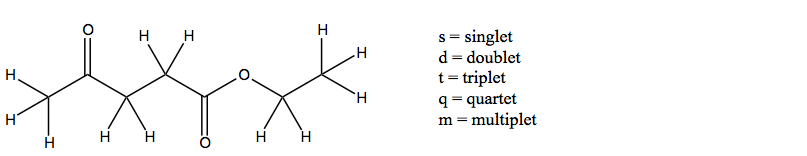# Problem: Indicate the splitting patterns expected for all of the equivalent sets of hydrogens in the molecule below. Assume that you observe the maximum possible number of peaks in each resonance. Also, assume that the splitting is negligible beyond 3 bonds.

###### FREE Expert Solution
96% (374 ratings)
###### Problem Details

Indicate the splitting patterns expected for all of the equivalent sets of hydrogens in the molecule below. Assume that you observe the maximum possible number of peaks in each resonance. Also, assume that the splitting is negligible beyond 3 bonds.What scientific concept do you need to know in order to solve this problem?

Our tutors have indicated that to solve this problem you will need to apply the 1H NMR: Spin-Splitting (N + 1) Rule concept. You can view video lessons to learn 1H NMR: Spin-Splitting (N + 1) Rule. Or if you need more 1H NMR: Spin-Splitting (N + 1) Rule practice, you can also practice 1H NMR: Spin-Splitting (N + 1) Rule practice problems.

What is the difficulty of this problem?

Our tutors rated the difficulty ofIndicate the splitting patterns expected for all of the equi...as medium difficulty.

How long does this problem take to solve?

Our expert Organic tutor, Jonathan took 4 minutes and 46 seconds to solve this problem. You can follow their steps in the video explanation above.

What professor is this problem relevant for?

Based on our data, we think this problem is relevant for Professor Neal's class at UT.